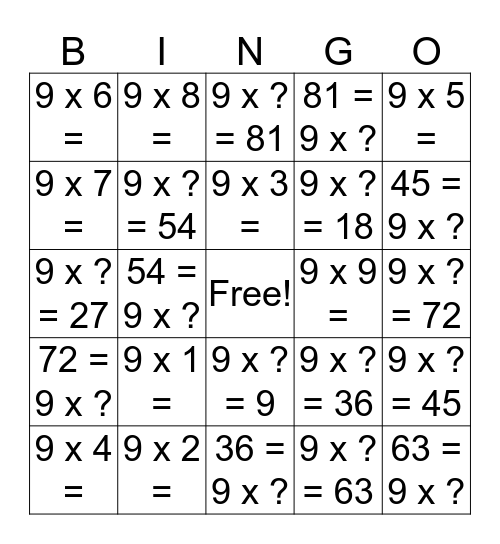9'sThis bingo card has a free space and 24 words: 9 x 1 =, 9 x 2 =, 9 x 3 =, 9 x 4 =, 9 x 5 =, 9 x 6 =, 9 x 7 =, 9 x 8 =, 9 x 9 =, 9 x ? = 9, 9 x ? = 18, 9 x ? = 27, 9 x ?= 36, 9 x ? = 45, 9 x ? = 54, 9 x ? = 63, 9 x ? = 72, 9 x ? = 81, 45 = 9 x ?, 54 = 9 x ?, 63 = 9 x ?, 72 = 9 x ?, 81 = 9 x ? and 36 = 9 x ?.

Play Online